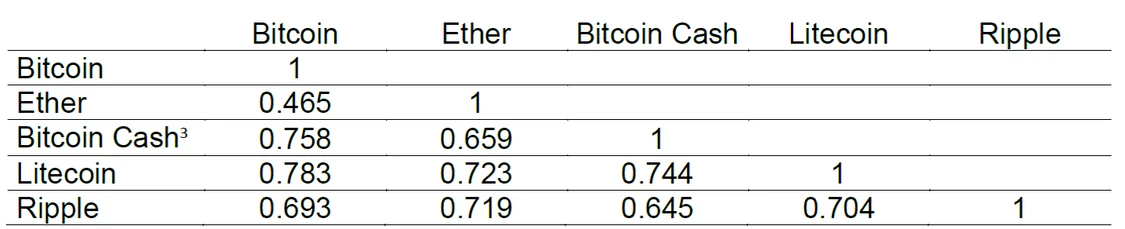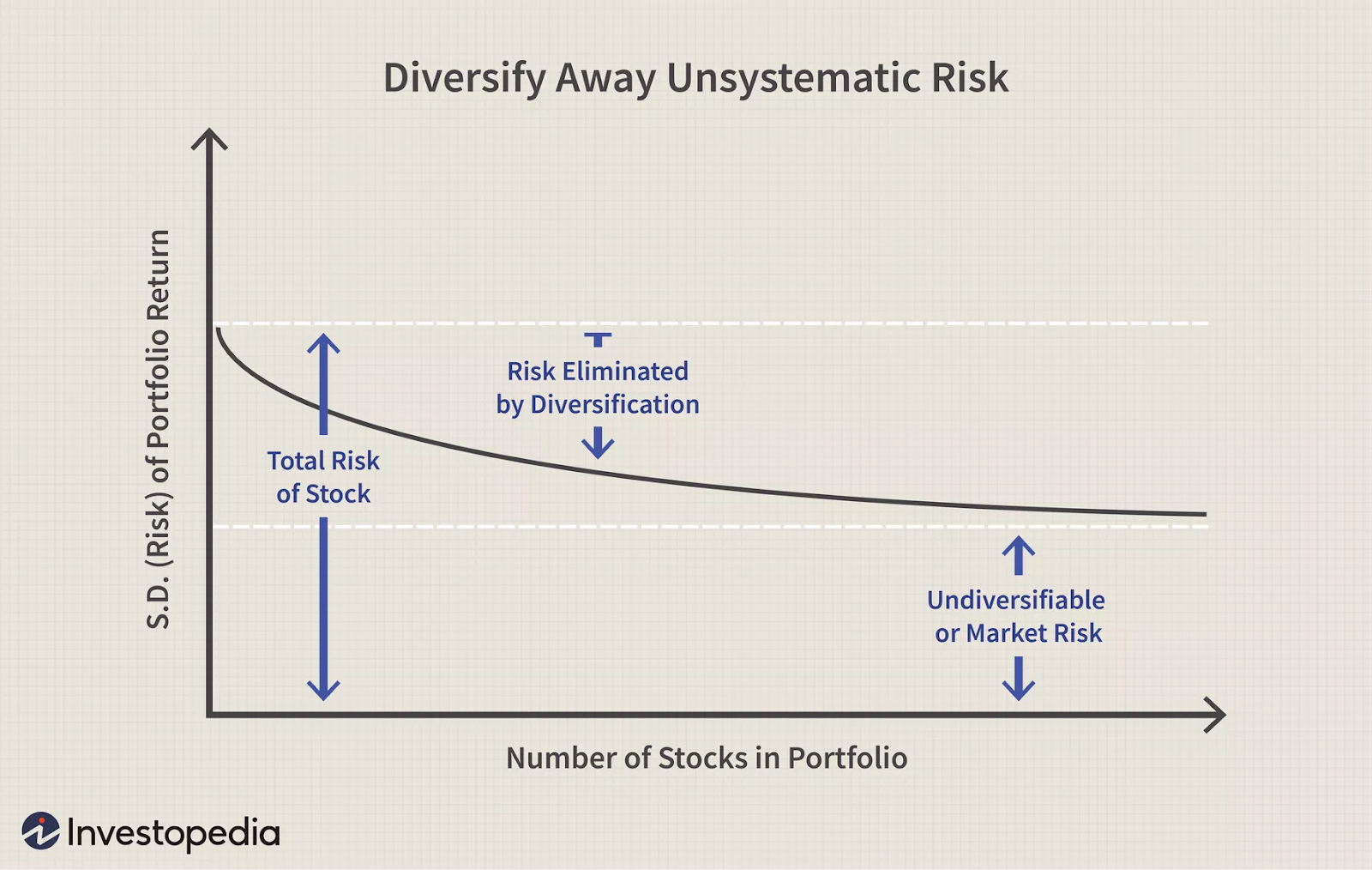Observations of the crypto market give the impression that “when Bitcoin sneezes, the cryptocurrency market catches a cold”. Technically, diversifying away risk in a crypto-only portfolio could be difficult. Creating a two-asset portfolio with highly correlated assets gives an investor a greater risk of losing more wealth. When two assets have a strong correlation coefficient they tend to move in the same direction. If  two assets in the same portfolio move in the same direction then your gains in wealth will be greater and your losses more severe. That could be the reason why investors try to create portfolios with negatively correlated assets. If one asset is declining in a portfolio consisting of two assets that are negatively correlated, then the other asset in the portfolio should be increasing. This should in effect diminish the maximum amount of wealth that can be lost in a portfolio.

Just from checking out the digital asset prices on a cryptocurrency exchange, one can see that they are highly correlated with one another.  If Bitcoin is in the red for the day, nearly every cryptocurrency on the homepage will be in the red, if Bitcoin is in the green, – so would be the others. That is why people say that “even the most unseaworthy boats will float when the tide rises”.Correlation of Bitcoin with other top cryptocurrencies

In particular, the Modern Portfolio Theory advocates diversification of securities and asset classes or the benefits of not putting all your eggs in one basket.

### Introduction to Modern Portfolio Theory (MPT)

Modern portfolio theory is based on the expected returns and the variance and covariance between the returns of assets. Investors try to form a portfolio that achieves the highest expected return but that does not exceed a given level of risk measured by the portfolio variance.

While the expected return of a portfolio of assets is the weighted average of the expected returns of its components, the variance of a portfolio of assets can be smaller than the average of the variance of its components because assets might move in opposite directions. If one asset gains while the other asset loses the overall volatility of the portfolio is smaller since the movements of the assets compensate each other.

The way to overcome this dilemma, MPT proposes, is through diversification, which refers to the spread of money across different asset classes and investments. According to MPT, an investor can hold a particular asset type or investment that is high in risk individually, but, when combined with several others of different types, the whole portfolio can be balanced in such a way that its risk is lower than the individual risk of underlying assets or investments.

Instead of focusing on the risk of each individual asset, Markowitz demonstrated that a diversified portfolio is less volatile than the total sum of its individual parts. While each asset itself might be quite volatile, the volatility of the entire portfolio can actually be quite low.

### Calculations

The expected return of the portfolio is calculated as a weighted sum of the individual assets’ returns. If a portfolio contained four equally weighted assets with expected returns of 4%, 6%, 10%, and 14%, the portfolio’s expected return would be:

`(4% x 25%) + (6% x 25%) + (10% x 25%) + (14% x 25%) = 8.5%`

The portfolio’s risk is a complicated function of the variances of each asset and the correlations of each pair of assets. To calculate the risk of a four-asset portfolio, an investor needs each of the four assets’ variances and six correlation values, since there are six possible two-asset combinations with four assets. Because of the asset correlations, the total portfolio risk, or standard deviation, is lower than what would be calculated by a weighted sum.

### Correlation

Diversification is a portfolio allocation strategy that aims to minimize idiosyncratic risk by holding assets that are not perfectly positively correlated. Correlation is simply the relationship that two variables share, and it is measured using the correlation coefficient, which lies between -1≤ρ≤1.

• A correlation coefficient of -1 demonstrates a perfect negative correlation between two assets. It means that a positive movement in one is associated with a negative movement in the other.
• A correlation coefficient of 1 demonstrates a perfect positive correlation. Both assets move in the same direction in response to market movements.

A perfect positive correlation between assets within a portfolio increases the standard deviation/risk of the portfolio. Diversification reduces idiosyncratic risk by holding a portfolio of assets that are not perfectly positively correlated.

For example, suppose a portfolio consists of assets A and B. The correlation coefficient for A and B is -0.9. This shows a strong negative correlation – a loss in A is likely to be offset by a gain in B. It is the advantage of owning a diversified portfolio.

### Kinds of Risk

Modern portfolio theory states that the risk for individual asset returns has two components:

Systematic Risk: This refers to market risks that cannot be reduced through diversification, or the possibility that the entire market and economy will show losses that negatively affect investments. It’s important to note that MPT does not claim to be able to moderate this type of risk, as it is inherent to an entire market or market segment.

Unsystematic Risk: Also called specific risk, unsystematic risk is specific to individual assets, meaning it can be diversified as you increase the number of assets in your portfolio.

For a well-diversified portfolio, the risk – or average deviation from the mean—of each asset contributes little to portfolio risk. Instead, it is the difference—or covariance—between individual asset’s levels of risk that determines overall portfolio risk. As a result, investors benefit from holding diversified portfolios instead of individual assets. As a result, assets with low correlations are generally good diversifiers in a portfolio whereas assets with negative correlations serve as a portfolio hedge.Systematic vs Unsystematic Risk

### The Efficient Frontier

While the benefits of diversification are clear, investors must determine the level of diversification that best suits them. This can be determined through what is called the Efficient Frontier, a graphical representation of all possible combinations of risky securities for an optimal level of return given a particular level of risk.

The Efficient Frontier is the cornerstone of MPT. You can think of it like this: Different cryptocurrencies will produce different returns. Say if you select three cryptos for your portfolio then the Efficient Frontier will represent the best combinations of these three cryptocurrencies. Every point on the Efficient Frontier represents the maximum expected return for a given level of risk.

Any portfolio that falls outside the Efficient Frontier is considered suboptimal for one of two reasons: it carries too much risk relative to its return, or too little return relative to its risk. A portfolio that lies below the Efficient Frontier doesn’t provide enough return when compared to the level of risk. Portfolios found to the right of the Efficient Frontier have a higher level of risk for the defined rate of return.

### How to diversify away risk in crypto portfolios?

Although digital assets are highly correlated, it is possible to diversify away risk in a crypto-only portfolio by adding more crypto assets to the portfolio. It is possible to diminish standard deviation when you move from a single-asset portfolio to a two-asset portfolio; from a two-asset portfolio to a three-asset portfolio, and then to four-asset portfolio and so on and so forth.

The reason you are able to diversify away risk in a crypto-only portfolio even though the crypto-assets are highly correlated could be because there are different types of risk.

1. Single asset risks: Risks of project failure, delisting from exchanges, ban from government, huge dump due to a major holder deciding to sell all his holdings one day.
2. Average industry growth: If you invest in just single or few assets, it is like playing the lottery. Your assets can perform differently – one could grow fast, and another could just make +10 percent and that is all. So, portfolio diversification gives you the opportunity to receive profit from the whole market growing and not depend just on having faith in one coin.
3. You can make different portfolios (for example high-risk, average, low risk) and receive profit that will be “averaged” on risk type.

Despite its volatility, Bitcoin has not exhibited a significant correlation with other traditional asset classes such as commodities, equities or fixed-income products since its creation in 2009 (with a median correlation coefficient with other asset classes below 0.10). Binance Research simulated different Bitcoin allocation techniques in existing diversified multi-asset portfolios. All simulated portfolios which included Bitcoin exhibited overall better risk-return profiles than traditional multi-asset class portfolios. These results show that Bitcoin provides active diversification benefits for all investors worldwide, following multi-asset strategies.

### Criticism of Modern Portfolio Theory (MPT)

Perhaps the most serious criticism of MPT is that it evaluates portfolios based on variance rather than downside risk. Two portfolios that have the same level of variance and returns are considered equally desirable under modern portfolio theory. One portfolio may have that variance because of frequent small losses. In contrast, the other could have that variance because of rare spectacular declines. Most investors would prefer frequent small losses, which would be easier to endure. Post-modern portfolio theory (PMPT) attempts to improve on modern portfolio theory by minimizing downside risk instead of variance.

The takeaway from this article is that spreading wealth over a number of assets instead of putting all into one could diversify away the idiosyncratic risk that is unique to a particular digital asset, and the more risk one is able to diversify away, the better situated he could be to protect himself against losses in the cryptocurrency portfolio.

Until next time!